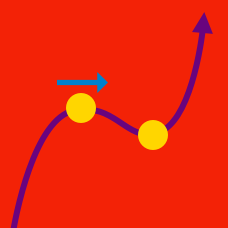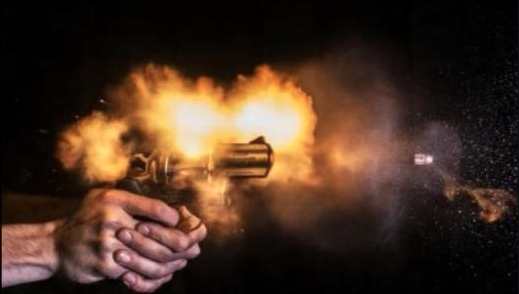Calculus

# Uniform Acceleration

A ball is currently $1000m$ above the ground and held at rest. It is dropped at $t = 0$, and experiences gravitational acceleration of $9.8 \, m / s^2$.

When $3$ seconds is passed, what is the height of the ball from the ground?

Object P with an initial velocity of $9\text{ m/s}$ starts to move eastward along a straight line under a constant acceleration of $8\text{ m/s}^2$ from point A. At the same time, another object Q starts to move eastward under a constant acceleration of $9\text{ m/s}^2$ from rest at point A. How far does object Q travel in meters when the two objects meet again?

An object with an initial velocity of $9\text{ m/s}$ moves along the $x$-axis with constant acceleration. After $8$ seconds, its velocity is $49\text{ m/s}.$ How far did it travel during the $8$ seconds in meters?The speed of a bullet is measured to be $640 \text{ m/s}$ as the bullet emerges from its $1.20 \text{ m}$ long barrel. Assuming a constant acceleration, find the time that the bullet spends in the barrel after it is fired.

A car that is initially traveling at $20\text{ m/s}$ accelerates uniformly in a straight line for $5$ seconds at a rate of $6\text{ m/s}^2.$ How far in meters does the car travel during the $5$ second period?

×